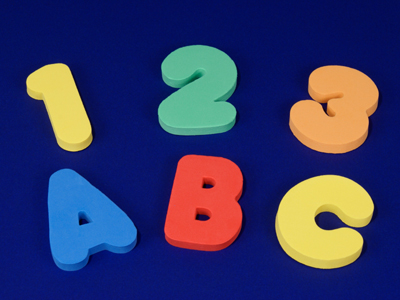Keep checking that you've got the correct letter-number match!

# Letters for Numbers 2

Letters for numbers means working out sums, so get your thinking cap on!

In this series of 11-plus verbal reasoning quizzes letters stand for numbers. Work out the correct answer to each sum and choose the correct answer from the four choices available.

The key to these questions is to practise, practise, practise. And when you're done practising, do a little more! If you're not sure what you are supposed to do, have a thorough read of the example given below.

Enjoy the second of this series and watch out for tricky answers! There's even a little bit of Pythagoras' theorem in this quiz. Don't worry, you will learn all about it next year at secondary school. For now, enjoy Letters for Numbers 2.

Please note that these questions assume you have not yet learned BODMAS, so if you have, please don't use BODMAS!

Example: What is the answer to the sum below, written as a letter?

A = 5, B = 14, C = 12, D = 13, E = 3
A + C - E = ?

A
B
C
E

Answer: The correct answer is B. If we translate all the letters into numbers, our new sum is 5 + 12 - 3, which = 14. 14 = B.
1.
What is the answer to the sum, written as a letter?

V = 32, E = 5, R = 18, Y = 25
E x E + Y - R
V
E
R
Y
If we translate all the letters into numbers, our new sum is 5 x 5 + 25 - 18, which = 32. 32 = V. Interesting number alert! The 5 times tables always end in 5 or 0. 5, 10, 15, 20, 25
2.
What is the answer to the sum, written as a letter?

C = 3, O = 4, D = 5, E = 25
C x C + (O x O) ÷ D
C
O
D
E
If we translate all the letters into numbers, our new sum is 3 x 3 + 4 x 4 ÷ 5, which = 5. 5 = D. Interesting number alert! Squaring the numbers 3 and 4 (9 and 16) and adding them, gives you the square of 5. So 32 + 42 = 52
3.
What is the answer to the sum, written as a letter?

T = 11, I = 99, N = 9, Y = 1
I ÷ T ÷ N x Y
T
I
N
Y
If we translate all the letters into numbers, our new sum is 99 ÷ 11 ÷ 9 x 1, which = 1. 1 = Y. Interesting number alert! The 11 times tables (up to 11 x 11) are all palindromes (they read the same forwards and backwards)
4.
What is the answer to the sum, written as a letter?

S = 22, C = 27, U = 45, N = 5
U ÷ (C - S) x N
S
C
U
N
If we translate all the letters into numbers, our new sum is 45 ÷ (27 - 22) x 5, which = 45. 45 = U
5.
What is the answer to the sum, written as a letter?

H = 3, A = 12, R = 15, D = 2
H + A x D ÷ R
H
A
R
D
If we translate all the letters into numbers, our new sum is 3 + 12 x 2 ÷ 15, which = 2. 2 = U
6.
What is the answer to the sum, written as a letter?

B = 20, U = 4, L = 32, K = 16
L ÷ U + K - B
B
U
L
K
If we translate all the letters into numbers, our new sum is 32 ÷ 4 + 16 - B , which = 4. 4 = U. Interesting number alert! 4 is a very important number in our culture. We have four seasons in a year and four school terms in a school year
7.
What is the answer to the sum, written as a letter?

P = 3, A = 27, L = 6, E = 9
P x E ÷ A x L
P
A
L
E
If we translate all the letters into numbers, our new sum is 3 x 9 ÷ 27 x 6, which = 6. 6 = L
8.
What is the answer to the sum, written as a letter?

M = 5, I = 3, L = 2 , K = 15
K ÷ M + L
M
I
L
K
If we translate all the letters into numbers, our new sum is 15 ÷ 5 + 2, which = 5. 5 = M. Interesting number alert! 2 and 5 are the only prime numbers that end with a 2 or a five
9.
What is the answer to the sum, written as a letter?

B = 5, A = 8, I = 3, T = 6
I + A - T + I
B
A
I
T
If we translate all the letters into numbers, our new sum is 3 + 8 - 6 + 3, which = 8. 8 = A
10.
What is the answer to the sum, written as a letter?

T = 8, Y = 4, R = 12 , E = 2
T x Y ÷ E - R
T
Y
R
E
If we translate all the letters into numbers, our new sum is 8 x 4 ÷ 2 - 12, which = 4. 4 = Y. Interesting number alert! 4 is the only number where the letters that spell it is equal to its value
Author:  Stephen O'Hara